Start typing, then use the up and down arrows to select an option from the list.Jules Bruno
220views
5
Hey, guys, in this new video, we're gonna put to practice some of the concepts we learned dealing with acids and bases. How doe identify them. And are they weak, or are they strong? So in this first example, it says, classify each of the following as a strong acid, a weak acid, a strong base or a week base. So remember, this is what we're gonna do. This is the protocol to follow when figuring out if something is an acid or base. First, we're gonna ask ourselves some important questions. First, let's identify if it's a base. We're going to say one. Does it have a metal for the first one? It doesn't have a medal to Doesn't have a negative charge anywhere. We don't see a negative charge. Three. Is it gonna mean remember, And a mean has H and N or H C and N doesn't have those lineups, so it's not in a mean. So that means they could not possibly be a base, so that means it's an acid. Is that a binary acid, or is it an oxy acid? If it has oxygen in it, it's a oxy acid, so we know it's not a base. So it has to be an oxy acid because of these oxygen's. We know it's an oxy acid, so let's just do the rules to determine if it's weak or strong. So remember, we look at the number of oxygen's we have to oxygen's minus two hydrogen, leaving us with nothing left. Remember, we need a minimum of two oxygen's left if we don't have that minimum. Couldn't be a strong acid, so this would be a week. Oxy acid. Next, let's go to C. We have H two N N H to ask ourselves the question. Does it have a metal? No. Does it have a negative charge? No. Is it in a mean Remember A means have H and end just like that compound. It has only H and N. Therefore, it isn't a mean. All you say now is Is it positive or is it neutral? It's a neutral, mean and neutral means are weak bases. So this particular week basis called hydrazine to so here. We're going to say this is a week base because it's a neutral amine. Next one be. Does it have a metal? No. Does it have a negative charge? No. Is it in a mean? It has a ches. It has carbon and it has a nitrogen, so it's definitely a mean. Now we just ask ourselves, Is it positive or is it neutral? Since it's positively charged and they will tell you? Since it's positively charged, it's in a mean it's a positive. I mean, so it must be a weak acid. And indeed, the last one. Does it have a medal? No. Does it have a negative charge? No. Is it in a mean? Does it have a Chand N? Nope. Does it have HC N? Nope. So it's an acid. If it has oxygen, it's an oxy acid. Here it has oxygen, so it's definitely an oxy acid. Let's do the math. Three. Oxygen's minus one hydrogen gives us to Oxygen's left. Definitely a strong oxy acid. So that's the protocol I want you guys to follow. We have more practice to do. I want you guys to attempt to do practice. Question one. Ask yourselves the questions. Does it have a medal? Does it have a negative charge? If it is it in a mean If Noda all three, then it's an acid. If it has no oxygen. It's a binary. If it does have oxygen, it's an oxy. And if we go back to bases, if it has a metal, remember, if it has a metal, what are the four ions it has to be connected to? To be a strong base. Say that to yourself if it if it's negative, Ion is not one of those four, um, ions that make it a strong base. Then it's a week base. So guys attempted to practice. Question went on your own. Come back, click on the explanation button and see what the answers are and how I determine them. Good luck.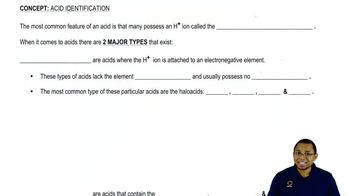05:07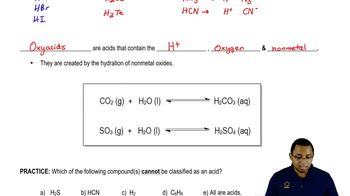02:36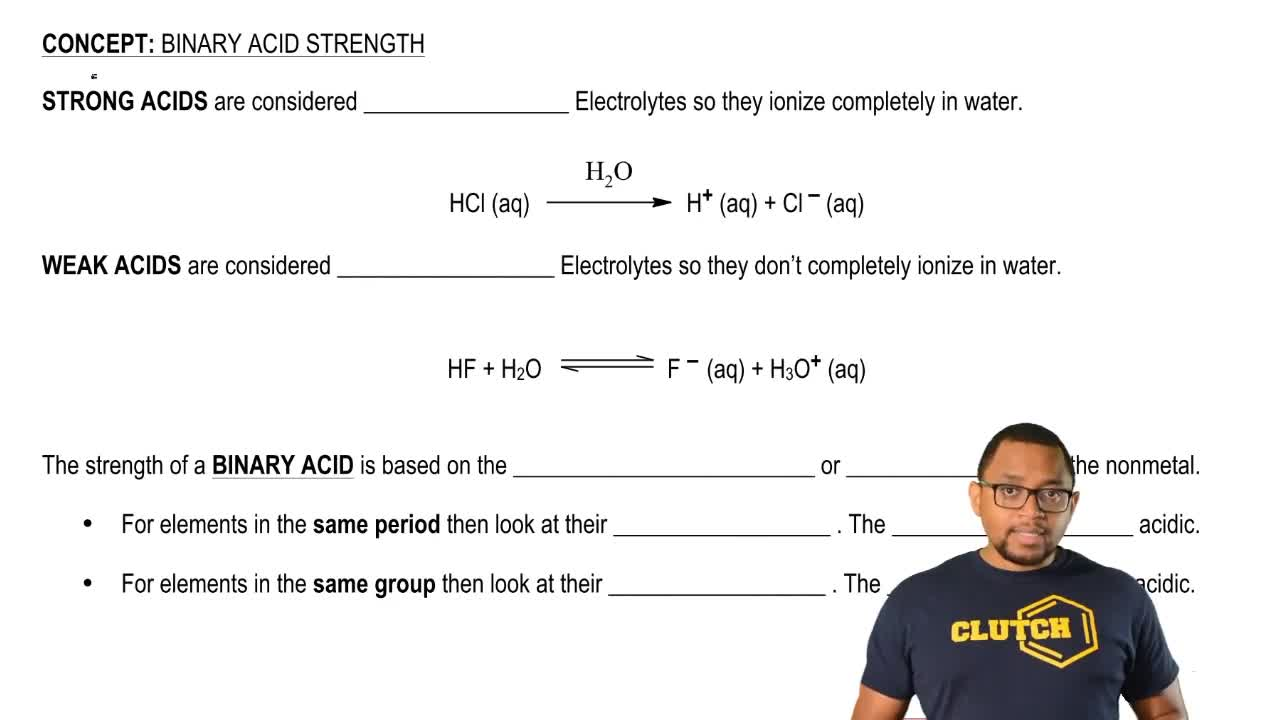02:04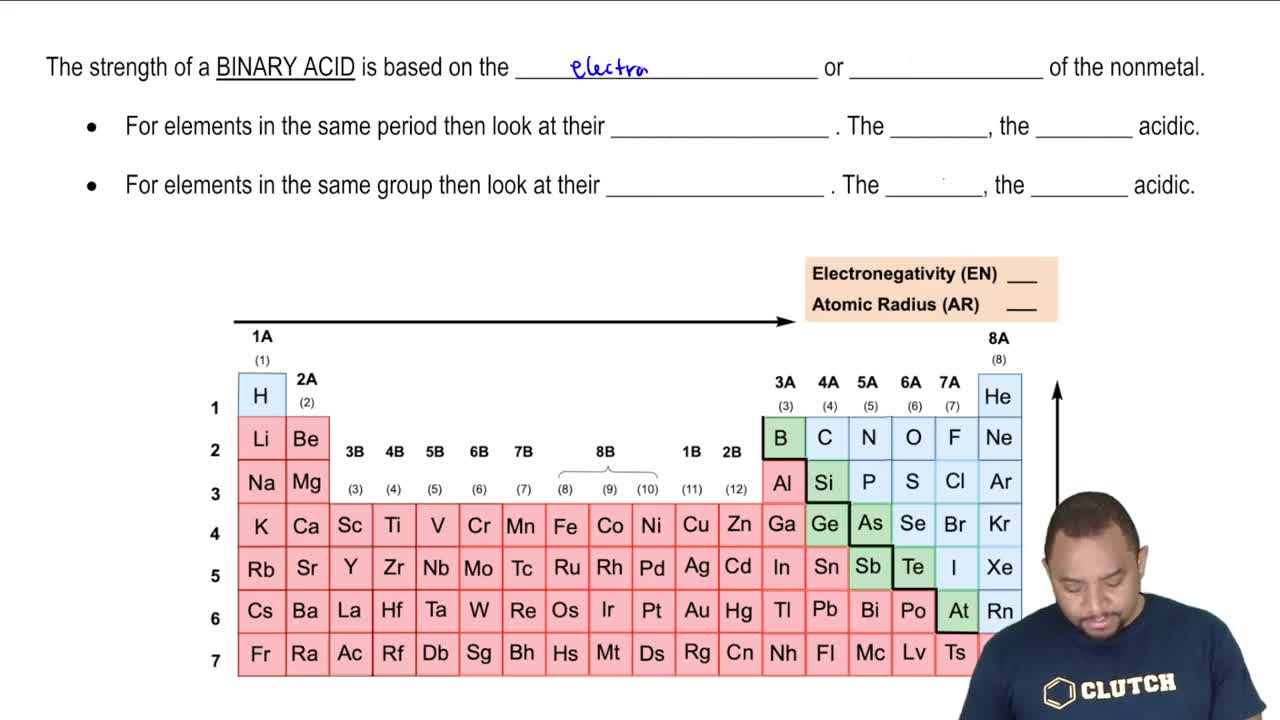05:05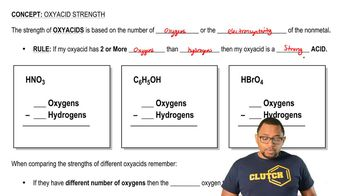02:08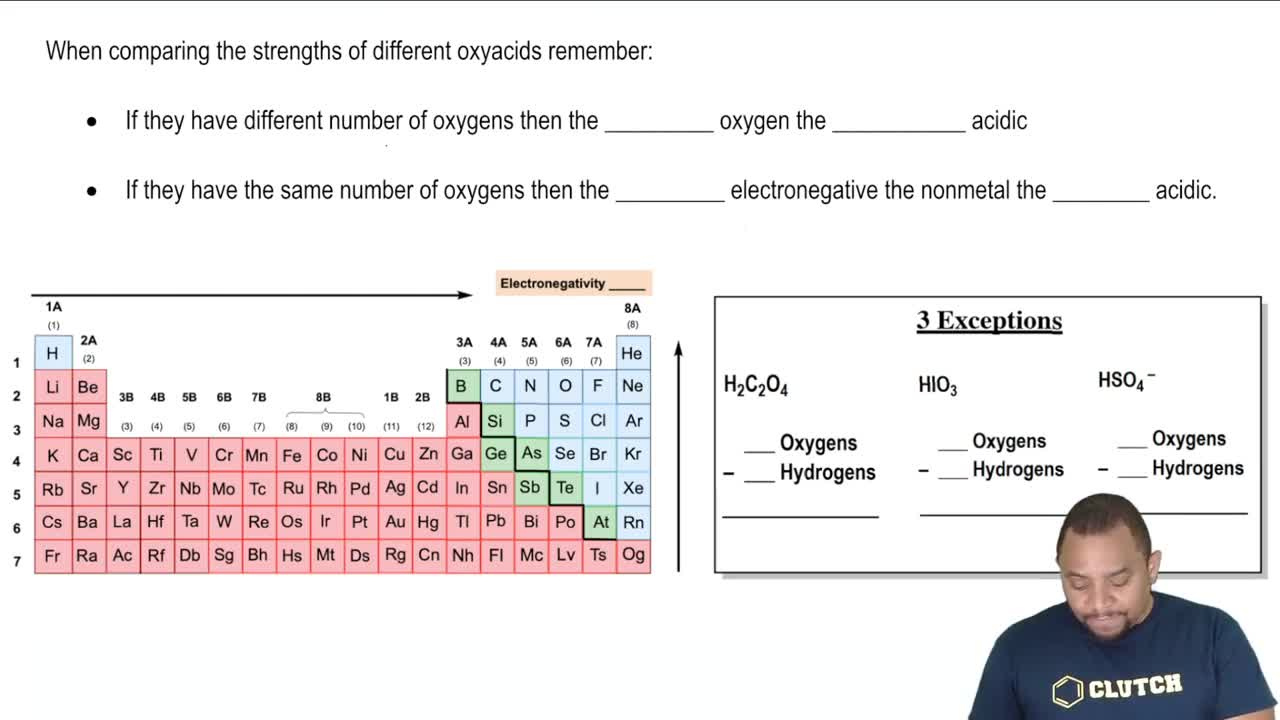03:53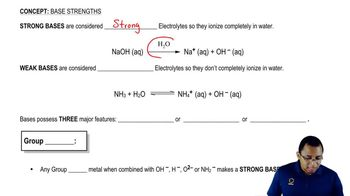02:06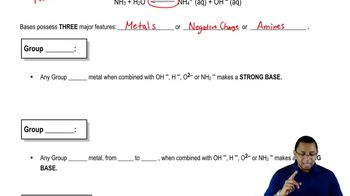02:53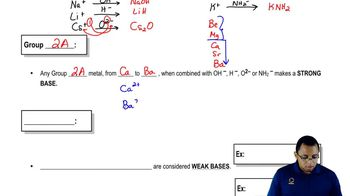02:41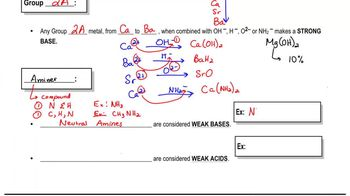03:22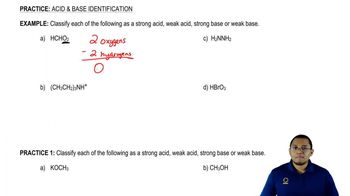04:29